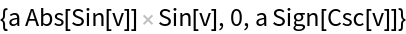Function Repository Resource:

# SecondFundamentalFormCoefficients

Compute the coefficients of the second fundamental form

Contributed by: Alfred Gray
 ResourceFunction["SecondFundamentalFormCoefficients"][s,{u,v}] computes the coefficients of the second fundamental form of surface s parametrized by u,v.

## Details and Options

The second fundamental form (or shape tensor) of a regular surface is a symmetric bilinear form on the tangent space in the three-dimensional Euclidean space.

## Examples

### Basic Examples (2)

Get the second fundamental form of a sphere:

 In:=Out=In:=Out=Enrique Zeleny

## Version History

• 1.0.0 – 01 April 2020